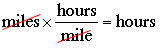SEARCH HOMEMath Central Quandaries & QueriesQuestion from Pauline, a student: During the first part of a trip, a canoeist travels 100 miles at a certain speed. The canoeist travels 25 miles on the second part of the trip at a speed 5 mph slower. The total time for the trip is 5 hrs. What was the speed on each part of the trip?Hi Pauline,

Suppose the canoeist travels for the first leg of the trip at s miles per hour then she travels the second leg at s - 5 miles per hour. You want the time for each leg since you know the sum of these two times is 5 hours. The units tell you what to do.

In the first leg you have distance = 100 miles, speed = s miles per hour and you want time in hours.Thus on the first leg

100 miles × 1/s hours/mile = 100/s hours

How long did the second leg take?

The sum of the two times is 5 hours. Solve for s.